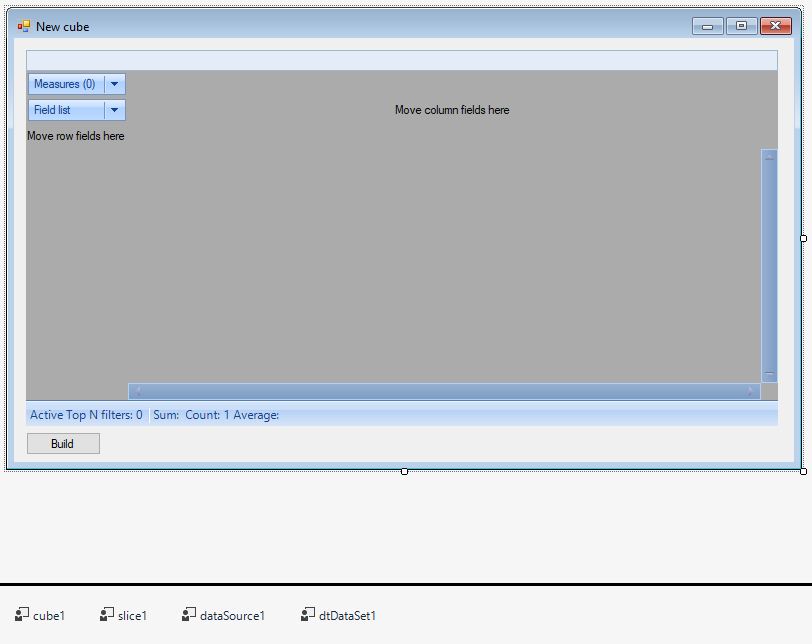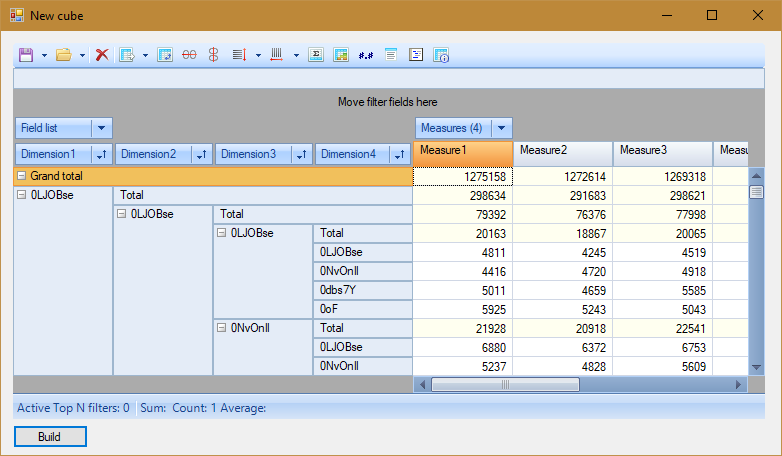How to create new cube in FastCube.NetDmitriy Fedyashov

In this article, we'll look at how to create a cube in FastCube.Net using code in a custom application. This question will appear to you right away, when you start working with this library. Well, I hope today we will clarify this issue.

The cube file can contain both data and its’ representation. Let's look at the whole chain of the cube creation.

Create a Windows Forms project. In References, you need to add links to the libraries: FastReport, FastReport.Bars, FastReport.Olap.

Add the following controls to the form from the toolbar:

dataSource, dtDataSet, cube, slice, sliceGrid, button.In the dataSource1 properties, we find the DataSet and select the available dtDataSet1 for it.

In the properties of the cube1 control, we select the value dataSource1 for the DataSource. And for the SoutceType property, the value is DataSource

In the slice1 properties, select the cube1 value for the Cube property.

Adjust the size of sliceGrid1. In its properties, select the slice1 value for Slice.

Now go to the form code.

First of all, let's add libraries to the Using section:

 ```1 2 3 4 5 6 ``` ```using System; using System.Collections.Generic; using System.Data; using System.Linq; using System.Windows.Forms; using FastReport.Olap.Slice;```

Add the following variables and objects to the class:

 ```1 2 3 4 5 6 7 ``` ``` const string chars = "ABCDEFGHIJKLMNOPQRSTUVWXYZabcdefghijklmnopqrstuvwxyz0123456789"; //symbol set private Random rnd = new Random(); //randomizer private DataTable dataTable = null; //data table object private int Dims = 4; //dimensions count private int Uniques = 500; //count of unique random values private int Measures = 4; //measures count private int Records = 10000; //table records count```

Here it is necessary to clarify that we will fill the table with fake data, just to demonstrate the possibility of software data generation. In addition to the DataTable, we can use a database or stream to retrieve data.

Create a DataTable and fill it with random data:

 ```1 2 3 4 5 6 7 8 9 10 11 12 13 14 15 16 17 18 19 20 21 22 23 24 25 26 27 28 29 30 31 32 33 34 35 36 37 38 39 40 41 42 43 44 45 46 47 48 49 50 51 52 53 54 55 56 57 58 ``` ```// The method of a random string generation private string GetNewStr(int len) { len = 1 + rnd.Next(len - 1); char[] resChars = new char[len]; for (int i = 0; i < resChars.Length; i++) { resChars[i] = chars[rnd.Next(chars.Length)]; } return new string(resChars); }   // The method of adding fields to a table private DataTable CreateDS() { DataTable dt = new DataTable("Test"); for (int i = 0; i < Dims; i++) dt.Columns.Add("Dimension" + (i + 1), typeof(string)); for (int i = 0; i < Measures; i++) dt.Columns.Add("Measure" + (i + 1), typeof(int)); return dt; }   // Method of filling the table with data private DataTable BuildDS() { DataTable dt = CreateDS();   int maxUniques = Uniques; SortedSet randomStrings = new SortedSet(); // Generating Unique Values while (randomStrings.Count < maxUniques) { randomStrings.Add(GetNewStr(10)); }   int dimCount = Dims; int mesCount = Measures;   object[] values = new object[dimCount + mesCount]; // Filling a table with data for (int i = 0; i < Records; i++) { for (int j = 0; j < dimCount; j++) { if (i < maxUniques) values[j] = randomStrings.ElementAt(i); else values[j] = randomStrings.ElementAt(rnd.Next(dimCount)); } for (int j = 0; j < mesCount; j++) { values[j + dimCount] = rnd.Next(255); } dt.Rows.Add(values); } return dt; }```

So, we have prepared a data source. I'll remind you that you can use a table or view from the database, or a data stream.

Now, create the OnClick event handler for our only button on the form. And write the following code:

 ```1 2 3 4 5 6 7 8 9 10 11 12 13 14 15 16 17 18 19 20 21 22 23 24 25 26 27 28 29 30 31 32 33 34 35 36 37 38 39 40 41 42 43 44 ``` ```private void button1_Click(object sender, EventArgs e) { // Add the generated table with the data in the DataSet cube1.Close(); DataTable dt = BuildDS(); if (dataTable != null) { dataTable.Dispose(); dataTable = null; } dataTable = dt;   dtDataSet1.DataTable = dataTable; dataSource1.DeleteFields(); cube1.Open(); dtDataSet1.DataTable = null; //Update slice slice1.BeginUpdate(); //Add fields into the containers for (int i = 0; i < Dims; i++) { slice1.YAxisContainer.AddSliceField(slice1.SliceFields.GetFieldByIndex(i)); } //Add empty measures container onto X-axis slice1.XAxisContainer.AddMeasuresField(); //Add measures into the measures container for (int i = 0; i < Measures; i++) { slice1.MeasuresContainer.AddMeasure(new MeasureField( slice1, FastReport.Olap.Types.AggregateFunction.Sum, slice1.SliceFields.GetFieldByIndex(i + Dims), null, null, "Measure" + (i + 1), "Measure" + (i + 1), false )); }   slice1.EndUpdate(); //Save cube into a file cube1.Save("J:/Program Files (x86)/FastReports/FastCube.Net Professional/Demos/C#/test.mdc"); }```

That's all. Dimensions and measures are stored in separate containers. First we filled the YAxisContainer with dimensions. Then, an empty list of measures was added to XAxisContainer. And only then began to fill it with measures. This order is important for the correct display of data.

To make it clearer wherewith we fill out the list of measures, I'll give the signature of the constructor of the class MeasureField:

public MeasureField(Slice slice, AggregateFunction aggregateFunction, SliceField baseSliceField, SliceField distinctSliceField, SliceField extraSliceField, string name, string caption, bool distinct);

In the final line of our code, we store the cube in the specified file.

Now start the application and create a new cube using the buttons:As you can see, it's quite easy to create a cube in the application code. It is more difficult to prepare data for it. But we'll talk about the data for the cube in another article.

Similar articles:

back